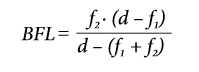+55 11 2839-3209

## UVFS Simple Telescope Kit

Simple lenses are subject to optical aberrations. In many cases these aberrations can be compensated for to a great extent by using a combination of simple lenses with complementary aberrations. A compound lens is a collection of simple lenses of different shapes and made of materials of different refractive indices, arranged one after the other with a common axis.

If two thin lenses are separated in air by some distance d (where d is smaller than the focal length of the first lens), the focal length for the combined system is given byThe distance from the second lens to the focal point of the combined lenses is called the back focal length (BFL). If the separation distance is equal to the sum of the focal lengths (d = f1 + f2), the combined focal length and BFL are infinite.If the separation distance is equal to the sum of the focal lengths (d=f1+f2), the combined focal length and BFL are infinite. This corresponds to a pair of lenses that transform a parallel (collimated) beam into another collimated beam. This type of system is called an afocal system, since it produces no net convergence or divergence of the beam. Two lenses at this separation form the simplest type of optical telescope. Although the system does not alter the divergence of a collimated beam, it does alter the width of the beam. The magnification of such a telescope is given bywhich is the ratio of the input beam width to the output beam width. Note the sign convention: a telescope with two convex lenses (f1 > 0, f2 > 0) produces a negative magnification, indicating an inverted image. A concave plus a convex lens (f1 < 0 < f2) produces a positive magnification and the image is upright.